# Inversion semi-group

inverse semi-group

A semi-group in which any elementpossesses a unique inverse element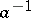(see Regular element). This property of a semi-groupis equivalent to each of the following properties:is a regular semi-group and any two of its idempotents commute (thus the set of all idempotents of an inverse semi-group is a semi-lattice, see Idempotents, semi-group of); each left or right principal ideal ofhas a unique generating idempotent. Every group is an inverse semi-group; groups are the only inverse semi-groups with a unique idempotent. An important role in the study of inverse semi-groups is played by the following natural partial order relationon an arbitrary inverse semi-group: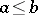if and only if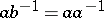(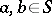). On the semi-lattice of idempotents of an inverse semi-group this relation is the same as the natural partial order of this semi-lattice (see Idempotent). A semi-lattice of inverse semi-groups (see Band of semi-groups) is an inverse semi-group. The translation hull of an inverse semi-group (see Translations of semi-groups) is also an inverse semi-group . Every congruence on an inverse semi-group is determined by the classes containing idempotents.

Let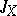be the set of all one-to-one partial transformations of a set(including the "empty transformation" , taking the empty set to itself). Thenis an inverse semi-group with respect to the operation of superposition, called the symmetric inverse semi-group on. The following Wagner–Preston theorem is of fundamental importance: Any inverse semi-groupcan be isomorphically imbedded in the symmetric inverse semi-group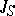.

The theory of inverse semi-groups is an important and deeply researched branch of the theory of semi-groups. Representations of inverse semi-groups by one-to-one partial transformations and matrices over a field have been studied (see ). Congruences on inverse semi-groups have been studied. Inverse semi-groups with finiteness conditions are being studied. Quite a number of important special types of inverse semi-groups have been singled out. The restrictions imposed on the majority of these bear the mark of simplicity in some sense (for example, bi-simplicity, see Simple semi-group), or relate to the semi-lattice of idempotents, or are combinations of both types. The restrictions onmay involve abstract properties ofas a semi-lattice (for example, thatbe a certain type of chain) or certain relative properties ofin the semi-group, in particular, the behaviour ofwith respect to certain congruences. There exists on any inverse semi-groupa least congruencewith the property thatis a group (the least group congruence), namely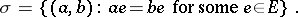An inverse semi-group is called proper ifconstitutes a-class. There exists on any inverse semi-groupa largest congruenceseparating idempotents, namely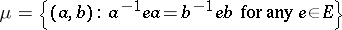andis contained in the relation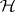(see Green equivalence relations); an inverse semi-group is called fundamental ifis the same as the equality relation. Quite a number of structure theorems have been obtained for inverse semi-groups of the above-mentioned types, and in many instances the description of inverse semi-groups is effected "modulo groups" ; the groups emerge as blocks of various structures in which semi-lattices, group homomorphisms, etc. also participate. Of this type, for example, are the typical descriptions of Clifford inverse semi-groups (see Clifford semi-group) and the completely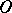-simple inverse semi-groups (see Brandt semi-group).

Inverse semi-groups can also be regarded as universal algebras with two operations: the binary operation of multiplication and the unary operation of taking the inverse element. A classification has been obtained of the monogenic (that is, generated by a single element) inverse semi-groups as universal algebras , . With respect to the above operations the class of all inverse semi-groups is a variety; it can be defined, for example, by the following system of identities :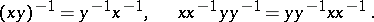How to Cite This Entry:
Inversion semi-group. Encyclopedia of Mathematics. URL: http://encyclopediaofmath.org/index.php?title=Inversion_semi-group&oldid=42685
This article was adapted from an original article by L.N. Shevrin (originator), which appeared in Encyclopedia of Mathematics - ISBN 1402006098. See original article